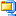In hibernate, we have many annotations. Each annotation has its importance to perform an operation. Likewise, @Formula is a hibernate annotation to calculate the dynamic value and assign that value to the property.

@Formula annotation takes the expression as a parameter, and at fetch time it will evaluate the expression and assigns an evaluated value to the property.

This @Formula parameter can be as complex as we want. That means, it may be a simple expression or it may be a complex query, this can be applied on top of the property.

In this tutorial, we are going to calculate the Employee total salary, using the @Formula annotation in hibernate.

## @Formula Hibernate Example :

To make the example as simple, we figure the total employee salary like below.

``Employee total salary = basic + conveyance + hra;``

Let’s implement this calculation by using @Formula annotation in hibernate. Project Structure:Required Dependencies :

pom.xml
``````<project xmlns="http://maven.apache.org/POM/4.0.0" xmlns:xsi="http://www.w3.org/2001/XMLSchema-instance" xsi:schemaLocation="http://maven.apache.org/POM/4.0.0 http://maven.apache.org/xsd/maven-4.0.0.xsd">
<modelVersion>4.0.0</modelVersion>
<groupId>org.springframework.samples</groupId>
<artifactId>Hibernate-Formula-Example</artifactId>
<version>0.0.1-SNAPSHOT</version>

<properties>

<!-- Generic properties -->
<java.version>1.6</java.version>
<project.build.sourceEncoding>UTF-8</project.build.sourceEncoding>
<project.reporting.outputEncoding>UTF-8</project.reporting.outputEncoding>

<!-- Spring -->
<spring-framework.version>3.2.3.RELEASE</spring-framework.version>

<!-- Hibernate / JPA -->
<hibernate.version>4.2.1.Final</hibernate.version>

<!-- Logging -->
<logback.version>1.0.13</logback.version>
<slf4j.version>1.7.5</slf4j.version>

<!-- Test -->
<junit.version>4.11</junit.version>

</properties>

<dependencies>
<!-- Hibernate -->
<dependency>
<groupId>org.hibernate</groupId>
<artifactId>hibernate-entitymanager</artifactId>
<version>\${hibernate.version}</version>
</dependency>
<!-- MySQL Driver -->
<dependency>
<groupId>mysql</groupId>
<artifactId>mysql-connector-java</artifactId>
<version>5.0.5</version>
</dependency>
</dependencies>
</project>``````

## Hibernate POJO :

Salaries.java

Salaries.java
``````package com.onlinetutorialspoint.bean;

import javax.persistence.Column;
import javax.persistence.Entity;
import javax.persistence.Id;
import javax.persistence.Table;

import org.hibernate.annotations.Formula;

@Entity
@Table(name = "salaries")
public class Salaries {
@Id
@Column(name = "empid")
private int empId;
@Column(name = "empname")
private String empName;
@Column(name = "basic")
private int basic;
@Column(name = "conveyance")
private int conveyance;
@Column(name = "hra")
private int hra;

@Formula(" basic + conveyance + hra ")
private float total;

public int getEmpId() {
return empId;
}

public void setEmpId(int empId) {
this.empId = empId;
}

public String getEmpName() {
return empName;
}

public void setEmpName(String empName) {
this.empName = empName;
}

public int getBasic() {
return basic;
}

public void setBasic(int basic) {
this.basic = basic;
}

public int getConveyance() {
return conveyance;
}

public void setConveyance(int conveyance) {
this.conveyance = conveyance;
}

public int getHra() {
return hra;
}

public void setHra(int hra) {
this.hra = hra;
}

public float getTotal() {
}

public void setTotal(float total) {
this.total = total;
}

}
``````

## Let’s Run the Example :

Main.java
``````import org.hibernate.HibernateException;
import org.hibernate.Session;
import org.hibernate.SessionFactory;
import org.hibernate.Transaction;
import org.hibernate.cfg.AnnotationConfiguration;

import com.onlinetutorialspoint.bean.Salaries;

public class Main {
private static final SessionFactory concreteSessionFactory;
static {
try {
concreteSessionFactory = new AnnotationConfiguration()
.configure().buildSessionFactory();
} catch (Throwable ex) {
throw new ExceptionInInitializerError(ex);
}
}
public static Session getSession()
throws HibernateException {
return concreteSessionFactory.openSession();
}

public static void main(String[] args) {
Session session=getSession();
Transaction tx = session.beginTransaction();
Salaries salaries = new Salaries();
salaries.setEmpName("Rahul");
salaries.setBasic(10000);
salaries.setConveyance(3000);
salaries.setHra(7000);
session.save(salaries);
tx.commit();

Salaries d=  (Salaries)session.get(Salaries.class,new Integer(1));

System.out.println("Employee Total Salary :"+d.getTotal());

}

}
``````

On the above example, we use session.get(Salaries.class, new Integer(1)) to get the Salaries details from hibernate cache or database.

Console
``````Hibernate: insert into salaries (basic, conveyance, empname, hra, empid) values (?, ?, ?, ?, ?)
Hibernate: select salaries0_.empid as empid1_0_0_, salaries0_.basic as basic2_0_0_, salaries0_.conveyance as conveyan3_0_0_, salaries0_.empname as empname4_0_0_, salaries0_.hra as hra5_0_0_,  salaries0_.basic + salaries0_.conveyance + salaries0_.hra  as formula0_0_ from salaries salaries0_ where salaries0_.empid=?
Employee Total Salary :20000.0``````

On the above example, we use @Formula annotation for simple expression. If we want to make this some more extent, we do.. Like below.

Console
``````@Formula("(select min(s.hra) from salaries s) ")
private float total;``````

If you change the code like above, you can get the minimum hra in salaries table.

Happy Learning 🙂

•Hibernate-Formula-Annotation-Example